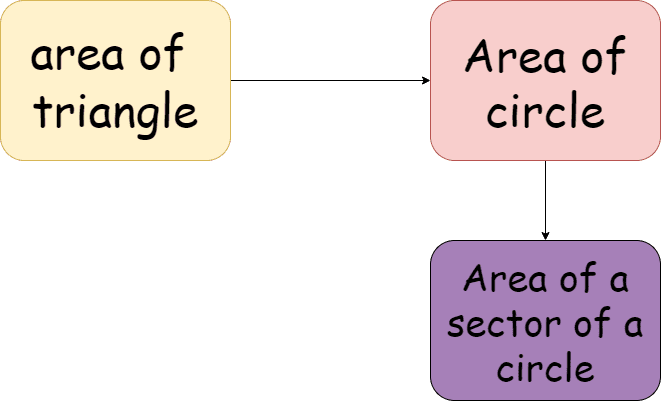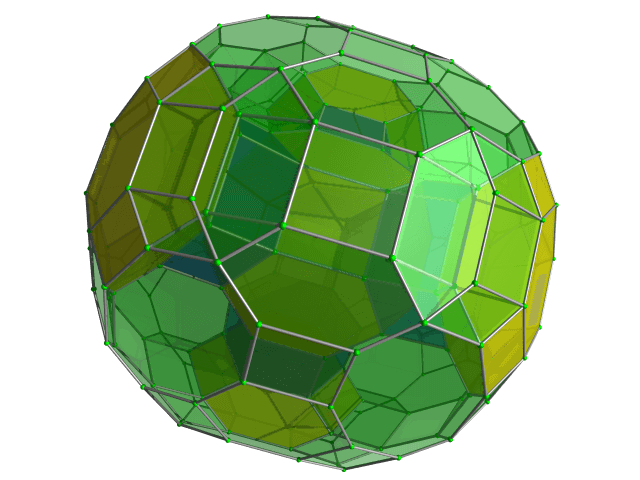Categories

## Application of Pythagoras Theorem | SMO, 2010 | Problem 22

Try this problem from the Singapore Mathematics Olympiad, SMO, 2010 based on the application of the Pythagoras Theorem.

## Application of Pythagoras Theorem- (SMO Test)

The figure below shows a circle with diameter AB. C ad D are points on the circle on the same side of AB such that BD bisects $\angle {CBA}$. The chords AC and BD intersect at E. It is given that AE = 169 cm and EC = 119 cm. If ED = x cm, find the value of x.

• 65
• 55
• 56
• 60

### Key Concepts

Circle

Pythagoras Theorem

2D – Geometry

Challenges and Thrills – Pre – College Mathematics

## Try with Hints

If you get stuck in this problem this is the first hint we can start with :

As BE intersect $\angle {CBA}$ we have $\frac {BC}{BA} = \frac {EC}{EA} = \frac {119}{169}$

Thus we can let BC = 119 y and BA = 169 y .

Since $\angle {BCA} = 90 ^\circ$.

Then try to do the rest of the problem ………………………………………………

If we want to continue from the last hint we have :

Apply Pythagoras Theorem ,

$AB ^2 = AC^2 + BC ^2$

$(169y)^2 = (169 + 119)^2 + (119y)^2$

$y^2 (169-119)(169+119) = (169+119)^2$

$y^2 = \frac {169+119}{169-119} = \frac {144}{25}$

$y = \frac {12}{5}$

In the last hint:

Hence , from triangle BCE , we have BE = $\sqrt{119^2 + (119y)^2} = 119 \times \frac {13}{5}$

Finally , note that $\triangle {ADE}$ and $\triangle {BCE}$ are similar , so we have

ED = $\frac {AE \times CE}{BE} = \frac {169 \times 119}{119 \times \frac {13}{5}} = 65$ cm .

Categories

## Triangle and Integer | PRMO 2019 | Question 28

Try this beautiful problem from the Pre-RMO, 2019 based on Circles,Triangle and largest integer.

## Circles, Triangle and largest Integer – PRMO 2019

Let ABC be a triangle with sides 51, 52, 53. Let D denote the incircle of triangle ABC. Draw tangents to D which are parallel to the sides ABC. let $r_1$. $r_2$, $r_3$ be the inradii of the three corner triangles so formed, find the largest integer that does not exceed $r_1+r_2+r_3$.

• is 107
• is 15
• is 840
• cannot be determined from the given information

### Key Concepts

Circles

Triangle

Largest Integers

PRMO, 2019, Question 28

Geometry Vol I to IV by Hall and Stevens

## Try with Hints

First hint

Let PQ be one of tangents parallel to BC and meet sides AB and AC at P and Q let PQ=x and BC=51

Second Hint

triangle ABC similar with triangle APQ then$\frac{x}{a}=\frac{r_1}{r}=\frac{s-a}{s}$ which is in same way for $\frac{y}{b}$ and $\frac{z}{c}$ then $\frac{r_1+r_2+r_3}{r}$=$\frac{x}{a}+\frac{y}{b}+\frac{z}{c}$=3-2=1

Final Step

then $r_1+r_2+r_3$=r and r by given condition of question =$(\frac{s(s-a)(s-b)(s-c)}{s})^\frac{1}{2}$=$(\frac{78(78-51)(78-52)(78-53)}{78})^\frac{1}{2}$=15.

Categories

## Cones and circle | AIME I, 2008 | Question 5

Try this beautiful problem from the American Invitational Mathematics Examination I, AIME I, 2008 based on Cones and circle.

## Cones and circle – AIME I, 2008

A right circular cone has base radius r and height h the cone lies on its side on a flat table. As the cone rolls on the surface of the table without slipping, the point where the cones base meets the table traces a circular arc centered at the point where the vertex touches the table. The cone first returns to its original position on the table after making 17 complete rotations. The value of $\frac{h}{r}$ can be written in the form $m{n}^\frac{1}{2}$ where m and n are positive integers and n in not divisible by the square of any prime, find m+n.

• is 107
• is 14
• is 840
• cannot be determined from the given information

### Key Concepts

Cones

Circles

Algebra

AIME I, 2008, Question 5

Geometry Vol I to IV by Hall and Stevens

## Try with Hints

First hint

The path is circle with radius =$({r}^{2}+{h}^{2})^\frac{1}{2}$ then length of path=$2\frac{22}{7}({r}^{2}+{h}^{2})^\frac{1}{2}$

Second Hint

length of path=17 times circumference of base then $({r}^{2}+{h}^{2})^\frac{1}{2}$=17r then ${h}^{2}=288{r}^{2}$

Final Step

then $\frac{h}{r}=12{(2)}^\frac{1}{2}$ then 12+2=14.

Categories

## Circles and points | HANOI 2018

Try this beautiful problem from HANOI, 2018 based on Circles and Points.

## Circles – HANOI 2018

The center of a circle and nine randomly selected points on this circle are colored in red. Every pair of those points is connected by a line segment, and every point of intersection of two line segments inside the circle is colored in red. Find the largest possible number of red points.

• is 224
• is 220
• is 228
• cannot be determined from the given information

### Key Concepts

Geometry

Circles

Combination

HANOI, 2018

Geometry Revisited by Coxeter

## Try with Hints

First hint

Remark that a convex quadrilateral has exactly one intersection which is the intersection of its two diagonals. Consider 9 points on the circle, which give at most $\frac{9!}{4!5!}$=126 intersections.

Second Hint

Considering the center and three points on the circle, there are at most $\frac{9!}{3!6!}$=84 intersections.

Final Step

So there are at most 126+84+10=220 red points.

Categories

## What is the area and perimeter of a circle?

A circle is a curve which maintains same distance from a fixed point called center.

The perimeter of a circle is the length of the curve and area of a circle is portion of a plane bounded by the curve.

## Try the problem

A ball with diameter 4 inches starts at point A to roll along the track shown. The track is comprised of 3 semicircular arcs whose radii are $R_1 = 100$ inches, $R_2 = 60$ inches, and $R_3 = 80$ inches, respectively. The ball always remains in contact with the track and does not slip. What is the distance the center of the ball travels over the course from A to B?

$\textbf{(A)}\ 238\pi \qquad \textbf{(B)}\ 240\pi \qquad \textbf{(C)}\ 260\pi \qquad \textbf{(D)}\ 280\pi \qquad \textbf{(E)}\ 500\pi$

AMC 8 2013 Problem 25

Geometry : Perimeter of a circle

7 out of 10

Mathematical Circles.

## Use some hints

First I want to give you the formula required.

You can clearly notice that we have to find the perimeters of all of the semicircles

The perimeter of a circle of radius $r$ unit can be obtained by the formula $2\pi r$. Then can you find perimeter of the semicircles ?!!!

So using the formula, the perimeters of

Semicircle 1 =$\frac{2\pi\times 100}{2}$ inches.

Semicircle 2 =$\frac{2\pi\times 60}{2}$ inches.

Semicircle 3 =$\frac{2\pi\times 80}{2}$ inches.

So the total path covered by the ball is

$\pi(100+60+80)=240\pi$ inches.

Is it the final answer??? Or have we ignored something ?

OK !!! please notice that they have asked for the distance covered by the center of the ball.

And the ball is of radius $2$ inches.

So for the $1^{st}$ and $3^{rd}$ semicircle : The center will roll along a semicircular path of radius $R_1-2$ and $R_3-2$.

See this image :And for the $2^{nd}$ semicircle : The center will roll along a semicircular path of radius $R_2+2$.

See the image below :So the length of the path covered by the center of the ball is

$[\pi(100-2)+\pi(60+2)+\pi(80-2)] \quad \text{inches} \\=\pi(98+62+78) \quad \text{inches}\\=238\pi \quad \text{inches}$.

Categories

## Competency in Focus: 2D Geometry (Areas related to circle)

This problem from American Mathematics Contest 8 (AMC 8, 2017) is based on calculation of areas related to circle. It is Question no. 25 of the AMC 8 2017 Problem series.

## First look at the knowledge graph:-## Next understand the problem

In the figure shown, $\overline{US}$ and $\overline{UT}$ are line segments each of length 2, and $m\angle TUS = 60^\circ$. Arcs $TR$ and $SR$ are each one-sixth of a circle with radius 2. What is the area of the region shown?$\textbf{(A) }3\sqrt{3}-\pi\qquad\textbf{(B) }4\sqrt{3}-\frac{4\pi}{3}\qquad\textbf{(C) }2\sqrt{3}\qquad\textbf{(D) }4\sqrt{3}-\frac{2\pi}{3}\qquad\textbf{(E) }4+\frac{4\pi}{3}$
##### Source of the problem
American Mathematical Contest 2017, AMC 8 Problem 25

### Finding the area of a triangle and sector of a circle. (Area related to circles)

5/10
##### Suggested Book

Do you really need a hint? Try it first!
C0nstruction : Let $X$ and $Y$ are the centres of the scetors $ST$ and $TR$ Now Let us join $SX$ and $TY$ What do you think? Will the points $U,S,\textbf{ and}\quad X$ be in a straightline?
$U,S,\textbf{ and}\quad X$ will be in a straight line because $\angle STU =60^{\circ}$ And angle of a  circle is $360$  i.e., $\angle SXR = \angle TYR = 60^{\circ}$ [Since sector($SXR$)=$\frac{1}{6}circle$] Then $UXY$ will make an equilateral triangle.
So after construction the figure will look like this :Therefore, The required area = Area of $\triangle UXY$ – $2 \times$ Area of the sector $SXR$.
Area of equilateral triangle $\triangle UXY= 4\sqrt{3}$ And the are of sector $SXR= \frac{2\pi}{3}$ ANS : $4\sqrt{3}-\frac{4\pi}{3}$
Area of an equilateral triangle =$\frac{a^2\sqrt{3}}{4}$ [where $a$ is a sied of the triangle] Area of a sector of a circle of angle $\theta$ = $\frac{\theta}{360}\pi r^2$ [where $r$ is the radius of the circle]

## AMC - AIME Program

AMC - AIME - USAMO Boot Camp for brilliant students. Use our exclusive one-on-one plus group class system to prepare for Math Olympiad## External Tangent | AMC 10A, 2018 | Problem 15

Try this beautiful Problem on geometry based on circle from AMC 10A, 2018. Problem-15. You may use sequential hints to solve the problem.

## Dice Problem | AMC 10A, 2014| Problem No 17

Try this beautiful Problem on Probability from AMC 10A, 2014. Problem-17, You may use sequential hints to solve the problem.

## Problem on Curve | AMC 10A, 2018 | Problem 21

Try this beautiful Problem on Co-ordinate geometry from AMC 10A, 2018. Problem-21, You may use sequential hints to solve the problem.

## Finding Greatest Integer | AMC 10A, 2018 | Problem No 14

Try this beautiful Problem on Algebra from AMC 10A, 2018. Problem-14, You may use sequential hints to solve the problem.

## Right-angled Triangle | AMC 10A, 2018 | Problem No 16

Try this beautiful Problem on triangle from AMC 10A, 2018. Problem-16. You may use sequential hints to solve the problem.

## Length of the crease | AMC 10A, 2018 | Problem No 13

Try this beautiful Problem on triangle from AMC 10A, 2018. Problem-13. You may use sequential hints to solve the problem.

## Right-angled shaped field | AMC 10A, 2018 | Problem No 23

Try this beautiful Problem on triangle from AMC 10A, 2018. Problem-23. You may use sequential hints to solve the problem.

## Area of region | AMC 10B, 2016| Problem No 21

Try this beautiful Problem on Geometry on Circle from AMC 10B, 2016. Problem-20. You may use sequential hints to solve the problem.

## Coin Toss Problem | AMC 10A, 2017| Problem No 18

Try this beautiful Problem on Probability from AMC 10A, 2017. Problem-18, You may use sequential hints to solve the problem.

## GCF & Rectangle | AMC 10A, 2016| Problem No 19

Try this beautiful Problem on Geometry on Rectangle from AMC 10A, 2010. Problem-19. You may use sequential hints to solve the problem.